# Maharashtra Board Class 11 Chemistry Important Questions Chapter 2 Introduction to Analytical Chemistry

Balbharti Maharashtra State Board 11th Chemistry Important Questions Chapter 2 Introduction to Analytical Chemistry Important Questions and Answers.

## Maharashtra State Board 11th Chemistry Important Questions Chapter 2 Introduction to Analytical Chemistry

Question 1.
Explain the statement: Analytical chemistry provides physical or chemical information about a sample.

• Analytical chemistry facilitates the investigation of the chemical composition of substances.
• It uses the instruments and methods to separate, identify and quantify a sample under study.

Thus, analytical chemistry provides chemical or physical information about a sample.

Question 2.
What is the difference between qualitative analysis and quantitative analysis?

1. Qualitative analysis deals with the detection of the presence or absence of elements in compounds and of chemical compounds in mixtures.
2. Quantitative analysis deals with the determination of the relative proportions of elements in compounds and of chemical compounds in mixtures.

Question 3.
Explain the importance of chemical analysis.

• Chemical analysis is one of the most important methods of monitoring the composition of raw materials, intermediates and finished products, and also the composition of air in streets and premises of industrial plants.
• In agriculture, chemical analysis is used to determine the composition of soils and fertilizers.
• In medicine, it is used to determine the composition of medicinal preparations.

Question 4.
Write a note on the applications of analytical chemistry.

• Analytical chemistry has applications in forensic science, engineering and industry.
• Analytical chemistry is also useful in the field of agriculture and pharmaceutical industry.
• Industrial process as a whole and the production of new kinds of materials are closely associated with analytical chemistry.Question 5.
What is semi-microanalysis?

• When the amount of a solid or liquid sample taken for analysis is a few grams, the analysis is called semi-microanalysis.
• It is of two types: qualitative and quantitative analysis.

Question 6.
What does classical qualitative analysis method include?
Classical qualitative analysis method includes separation and identification of compounds.

• Separations may be done by methods such as precipitation, extraction and distillation.
• Identification may be based on differences in colour, odour, melting point, boiling point, and reactivity.

Question 7.
Name two methods of classical quantitative analysis.

1. Volumetric analysis (Titrimetric analysis)
2. Gravimetric analysis (i.e., decomposition, precipitation)

Question 8.
What are the two stages involved in the chemical analysis of a sample?
The chemical analysis of a sample is carried out mainly in two stages: by the dry method and by the wet method. In dry method, the sample under test is not dissolved and in wet method, the sample under test is first dissolved and then analysed to determine its composition.

Question 9.
Explain: Qualitative analysis of organic compounds

• The majority of organic compounds are composed of a relatively small number of elements.
• The most important ones are: carbon, hydrogen, oxygen, nitrogen, sulphur, halogen, phosphorus.
• Elementary qualitative analysis is concerned with the detection of the presence of these elements.
• The identification of an organic compound involves tests such as detection of functional group, determination of melting/boiling points, etc.Question 10.
What does qualitative analysis of inorganic compounds involve?
The qualitative analysis of simple inorganic compounds involves detection and confirmation of cationic and anionic species (basic and acidic radical) in them.

Question 11.
Explain: Chemical methods of quantitative analysis

• Quantitative analysis of organic compounds involves methods such as determination of percentage of constituent elements, concentrations of a known compound in the given sample, etc.
• Quantitative analysis of simple inorganic compounds involves methods such as gravimetric analysis (i.e., decomposition, precipitation, etc.) and the titrimetric or volumetric analysis (i.e., progress of reaction between two solutions till its completion).
• The quantitative analytical methods involve measurement of quantities such as mass and volume using some equipment/apparatus such as weighing machine, burette, etc.

Question 12.
Why is accurate measurement crucial in science?

• The accuracy of measurement is of a great concern in analytical chemistry. This is because faulty equipment, poor data processing or human error can lead to inaccurate measurements. Also, there can be intrinsic errors in the analytical measurement.
• When measurements are not accurate, this provides incorrect data that can lead to wrong conclusions. For example, if a laboratory experiment requires a specific amount of a chemical, then measuring the wrong amount may result in an unsafe or unexpected outcome.
• Hence, the numerical data obtained experimentally are treated mathematically to reach some quantitative conclusion.
• Also, an analytical chemist has to know how to report the quantitative analytical data, indicating the extent of the accuracy of measurement, perform the mathematical operation and properly express the quantitative error in the result.

Question 13.
Why are scientific notations (exponential notations) used?
A chemist has to deal with numbers as large as 602,200,000,000,000,000,000,000 for the molecules of 2 g of hydrogen gas or as small as 0.00000000000000000000000166 g. that is, mass of a H atom. To avoid the writing of so many zeros in mathematical operations, scientific notations i.e. exponential notations are used.

Question 14.
How are numbers expressed in scientific notations (exponential notations)?
In scientific notations, numbers are expressed in the form of N × 10n, where ‘n’ is an exponent with positive or negative values and N can have a value between 1 to 10.
e.g. i. The number, 602,200,000,000,000,000,000,000 is expressed as 6.022 × 1023.
ii. The mass of a H atom, 0.00000000000000000000000166 g is expressed as 1.66 × 10-24 g.
iii. The number 123.546 is written as 1.23546 × 102.
iv. The number 0.00015 is written as 1.5 × 10-4.Question 15.
Express the following quantities in scientific notations (exponential notations).
i. 0.0345
ii . 0.08
iii. 653.00
iv. 34.768
i. 0.0345 = 3.45 × 10-2
ii. 0.08 = 8 × 10-2
iii. 653.00 = 6.5300 × 102
iv. 34.768 = 3.4768 × 101

Question 16.
Define: Accuracy of measurement
Nearness of the measured value to the true value is called the accuracy of measurement.

Question 17.
Explain with the help of a diagram how accuracy depends upon the sensitivity or least count of the measuring equipment.
A burette reading of 10.2 mL is as shown in the diagram below: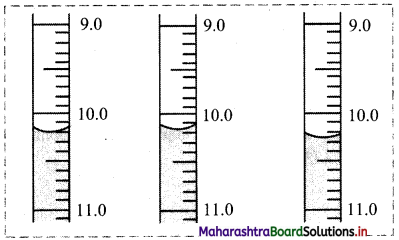• For all the three situations in the above figure, the reading would be noted is 10.2 mL.
• It means that there is an uncertainty about the digit appearing after the decimal point in the reading 10.2 mL because the least count of the burette is 0.1 mL.
• The meaning of the reading 10.2 mL is that the true value of the reading lies between 10.1 mL and 10.3 mL.
• This is indicated by writing 10.2 ± 0.1 mL.
• Here, the burette reading has an error of ± 0.1 mL.
• Smaller the error, higher is the accuracy.

Question 18.
How is absolute error calculated?
Absolute error is calculated by subtracting true value from observed value.
Absolute error = Observed value – Tme value

Question 19.
Explain the term: Relative error

1. Relative error is the ratio of an absolute error to the true value.
2. Relative error is generally a more useful quantity than absolute error.
3. Relative error is expressed as a percentage and can be calculated as follows:
Relative error = $$\frac{\text { Absolute error }}{\text { True value }} \times 100 \%$$Question 20.
Explain the term: Precision in measurement

• Multiple readings of the same quantity are noted to minimize the error.
• If the multiple readings of the same quantity match closely, they are said to have high precision.
• High precision implies reproducibility of the readings.
• High precision is a prerequisite for high accuracy.
• Precision is expressed in terms of deviation (i.e. absolute deviation and relative deviation).

Question 21.
Explain the following terms with respect to precise measurement:
i. Absolute deviation
ii. Mean absolute deviation
iii. Relative deviation
i. Absolute deviation: An absolute deviation is the modulus of the difference between an observed value and the arithmetic mean for the set of several measurements made in the same way. It is a measure of absolute error in the repeated observation. It is expressed as follows:
Absolute deviation = |Observed value – Mean|

ii. Mean absolute deviation: Arithmetic mean of all the absolute deviations is called the mean absolute deviation in the measurements.

iii. Relative deviation: The ratio of mean absolute deviation to its arithmetic mean is called relative deviation. It is expressed as follows:
Relative deviation = $$\frac{\text { Mean absolute deviation }}{\text { Mean }}$$ × 100%

Question 22.
Explain the need of significant figures in measurement.

• Uncertainty in measured value leads to uncertainty in calculated result.
• Uncertainty in a value is indicated by mentioning the number of significant figures in that value. e.g. Consider, the column reading 10.2 ± 0.1 mL recorded on a burette having the least count of 0.1 mL. Here, it is said that the last digit ‘2’ in the reading is uncertain, its uncertainty is ±0.1 mL. On the other hand, the figure ‘10’ is certain.
• The significant figures in a measurement or result are the number of digits known with certainty plus one uncertain digit.
• In a scientific experiment, a result is obtained by doing calculation in which values of a number of quantities measured with equipment of different least counts are used.

Question 23.
How many significant figures are present in the following measurements?
i. 4.065 m
ii. 0.32 g
iii. 57.98 cm3
iv. 0.02 s
v. 4.0 × 10-4 km
vi. 604.0820 kg
vii. 307.100 × 10-5 cm
i. 4
ii. 2
iii. 4
iv. 1
v. 2
vi. 7
vii. 6

Question 24.
How many significant figures are present in each of the following?
i. 45.0
ii. 0.001
iii. 2.10 × 10-8
iv. 340000
v. 0.0100
vi. 7890320
vii. 100.00
viii. 100
i. 3
ii. 1
iii. 3
iv. 2
v. 3
vi. 6
vii. 5
viii. 1Question 25.
State the rules used to round off a number to the required number of significant figures.
The following rules are used to round off a number to the required number of significant figures:

• If the digit following the last digit to be kept is less than five, the last digit is left unchanged, e.g. 46.32 rounded off to two significant figures is 46.
• If the digit following the last digit to be kept is five or more, the last digit to be kept is increased by one. e.g. 52.87 rounded to three significant figures is 52.9.

Question 26.
Round off each of the following to the number of significant digits indicated:
i. 1.223 to two digits
ii. 12.56 to three digits
iii. 122.17 to four digits
iv. 231.5 to three digits
i. 1.223 to two digits = 1.2
This is because the third digit is less than 5, so we drop it and all the other digits to its right.
ii. 12.56 to three digits = 12.6
This is because the fourth digit is greater than 5, so we drop it and add 1 to the third digit.
iii. 122.17 to four digits = 122.2
This is because the fifth digit is greater than 5, so we drop it and add 1 to the fourth digit.
iv. 231.5 to three digits = 232
This is because the fourth digit is 5, so we drop it and add 1 to the third digit.

Question 27.
Add 5.55 × 104 and 6.95 × 103 and express the result in scientific notation.
Solution:
To perform addition operation, first the numbers are written in such a way that they have the same exponent. The coefficients are then added.
(5.55 × 104) + (6.95 × 103)= (5.55 × 104) + (0.695 × 104)
= (5.55 +0.695) × 104
= 6.245 × 104

Question 28.
Add 1.77 × 102 and 2.23 × 103 and express the result in scientific notation.
Solution:
To perform addition operation, first the numbers are written in such a way that they have the same exponent. The coefficients are then added.
(1.77 × 102) + (2.23 × 103) = (0.177 × 103) + (2.23 × 103)
= (0.177 + 2.23) × 103
= 2.407 × 103

Question 29.
Subtract 5.8 × 10-3 from 3.5 × 10-2 and express result in scientific notation.
Solution:
To perform subtraction operation, first the numbers are written in such a way that they have the same exponent. Then subtraction of coefficients can be done.
(3.5 × 10-2)- (5.8 × 10-3) = (3.5 × 10-2) – (0.58 × 10-2)
= (3.5 – 0.58) × 10-2
= 2.92 × 10-2Question 30.
Subtract 6.90 × 10-5 from 5.11 × 10-4 and express the result in scientific notation.
Solution:
To perform subtraction operation, first the numbers are written in such a way that they have the same exponent. Then subtraction of coefficients can be done.
(5.11 × 10-4) – (6.90 × 10-5) = (5.11 × 10-4) – (0.690 × 10-4)
= (5.11 – 0.690) × 10-4
= 4.42 × 10-4

Question 31.
Perform following calculations and express results in scientific notations (exponential notations),
i. (1.5 × 10-6) – (5.8 × 10-7)
ii. (9.8 × 10-3) – (8.8 × 10-3)
iii. (6.5 × 10-8) – (5.5 × 10-9)
Solution:
To perform subtraction operation, first the numbers are written in such a way that they have the same exponent. Then subtraction of coefficients can be done.
i. (1.5 × 10-6) – (5.8 × 10-7) = (1.5 × 10-6) – (0.58 × 10-6)
= (1.5 – 0.58) × 10-6
= 0.92 × 10-6
= 9.2 × 10-7

ii. (9.8 × 10-3) – (8.8 × 10-3) = (9.8 – 8.8) × 10-3
= 1.0 × 10-3

iii. (6.5 × 10-8) – (5.0 × 10-9) = (6.5 × 10-8) – (0.50 × 10-8)
= (6.5 – 0.50) × 10-8
= 6.0 × 10-8

Question 32.
Multiply 5.6 × 105 and 6.9 × 108 and express result in scientific notation.
Solution:
(5.6 × 105) × (6.9 × 108) = (5.6 × 6.9) (105+8)
= 38.64 × 1013
= 3.864 × 1014

Question 33.
Multiply 9.8 × 10-2 and 2.5 × 10-6 and express result in scientific notation.
Solution:
(9.8 × 10-2) × (2.5 × 10-6) = (9.8 × 2.5) (10-2+(-6))
= (9.8 × 2.5) × (10-2-6)
= 24.5 × 10-8
= 2.45 × 10-7

Question 34.
Perform following calculations and express results in scientific notations (exponential notations).
i. (2.5 × 10-6) × (1.8 × 10-7)
ii. (4.5 × 10-3) × (1.8 × 103)
iii. (8.5 × 107) × (3.5 × 109)
Solution:
i. (2.5 × 10-6) × (1.8 × 10-7) = (2.5 × 1.8) (10-6+(-7))
= (2.5 × 1.8) × (10-6-7)
= 4.5 × 10-13

ii. (4.5 × 10-3) × (1.8 × 103) = (4.5 × 1.8) (10-3+3)
= (4.5 × 1.8) × (100)
= 8.1

iii. (8.5 × 107) × (3.5 × 109) = (8.5 × 3.5) (107+9)
= (8.5 × 3.5) × 1016
= 29.75 × 1016
= 2.975 × 1017
[Note: To express number in scientific notation, the number has to be greater than or equal to 10 or less than 1. The number, 8.1 is greater than 1, but less than 10 and hence, it cannot be expressed in scientific notation.]Question 35.
In laboratory experiment, 10 g potassium chlorate sample on decomposition gives following data: The sample contains 3.8 g of oxygen and the actual mass of oxygen in the quantity of potassium chlorate is 3.92 g. Calculate absolute error and relative error.
Solution:
The observed value is 3.8 g and accepted (true) value is 3.92 g.
i. Absolute error = Observed value – True value
= 3.8 – 3.92
= -0.12 g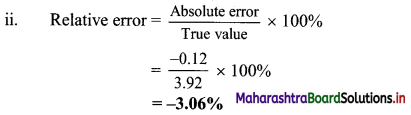[Note: The negative sign indicates that experimental result is lower than the true value.]
Ans: i. Absolute error = -0.12 g
ii. Relative error = -3.06%

Question 36.
12.15 g of magnesium gives 20.20 g of magnesium oxide on burning. The actual mass of magnesium oxide that should be produced is 20.15 g. Calculate absolute error and relative error.
Solution:
The observed value is 20.20 g and accepted (true) value is 20.15 g.
i. Absolute error = Observed value – True value
= 20.20 – 20.15
= 0.05 g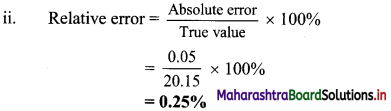Ans: i. Absolute error = 0.05 g
ii. Relative error = 0.25 %

Question 37.
The three identical samples of potassium chlorate are decomposed. The mass of oxygen is determined to be 3.87 g, 3.95 g and 3.89 g for the set. Calculate absolute deviation and relative deviation.
Solution:
Mean = $$\frac{3.87+3.95+3.89}{3}$$ = 3.90

 Sample Mass of oxygen Absolute deviation = | Observed value – Mean | 1 3.87 g 0.03 g 2 3.95 g 0.05 g 3 3.89 g 0.01 g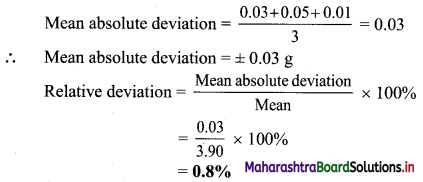Ans: i. Absolute deviation in each observation = 0.03 g, 0.05 g, 0.01 g
Relative deviation = 0.8%

Question 38.
In repeated measurements in volumetric analysis, the end-points were observed as 11.15 mL, 11.17 mL, 11.11 mL and 11.17 mL. Calculate mean absolute deviation and relative deviation.
Solution:
Mean = $$\frac{11.15+11.17+11.11+11.17}{4}$$ = 11.15

 Measurement End-point Absolute deviation = |Observed value – Mean| 1 11.15 mL 0 2 11.17 mL 0.02 mL 3 11.11 mL 0.04 mL 4 11.17 mL 0.02 mL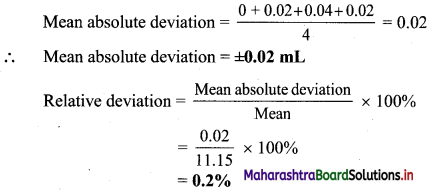Ans: i. Mean absolute deviation = ±0.02 mL
ii. Relative deviation = 0.2%

Question 39.
Calculate the mass percentages of H, P and O in phosphoric acid if atomic masses are H = 1, P = 31 and O = 16.
Solution:
Atomic mass of H = 1, P = 31 and 0=16
The mass percentage of hydrogen, phosphorus, oxygen in H3PO4
Formula: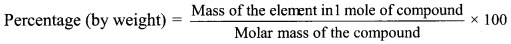Calculation: Molecular formula of phosphoric acid: H3PO4
∴ Molar mass of H3PO4 = 3 × (1) + 1 × (31) + 4 × (16)
= 3 + 31 + 64
= 98 g mol-1
Percentage of H = $$\frac {3}{98}$$ × 100 = 3.06%
Percentage of P = $$\frac {31}{98}$$ × 100 = 31.63%
Percentage of O = $$\frac {64}{98}$$ × 100 = 65.31%
Ans: Mass percentage of H, P and O in phosphoric acid are 3.06%, 31.63% and 65.31% respectively.Question 40.
Calculate the percentage composition of the elements in HNO3 (H = 1, N = 14, O = 16).
Solution:
Atomic mass of H = 1, N = 14 and O = 16
The mass percentage of H, N and O in HNO3
Formula: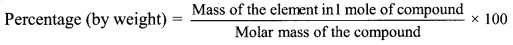Calculation: Molecular formula of HNO3
∴ Molar mass = 1 × (1) + 1 × (14) + 3 × (16) = 1 + 14 + 48 = 63 g mol-1
∴ Percentage of H = $$\frac {1}{63}$$ × 100 = 1.59%
Percentage of N = $$\frac {14}{63}$$ × 100 = 22.22%
Percentage of O = $$\frac {48}{63}$$ × 100 = 76.19%
Ans: Mass percentage of H, N and O in HNO3 are 1.59%, 22.22% and 76.19% respectively.

Question 41.
A compound contains 4.07% hydrogen, 24.27% carbon and 71.65% chlorine by mass. Its molar mass is 98.96 g mol-1. What is its empirical formula and molecular formula? Atomic masses of hydrogen, carbon and chlorine are 1.008,12.000 and 35.453 u, respectively
Solution:
Given: Percentage of H, C and Cl = 4.07% , 24.27% and 71.65% by mass respectively.
To find: Empirical formula and molecular formula
Calculation: Step I:
Check whether the sum of all the percentages is 100.
4.07 + 24.27 + 71.65 = 99.99 ≈ 100
Therefore, no need to consider presence of oxygen atom in the molecule.

Step II:
Conversion of mass percent to grams. Since we are having mass percent, it is convenient to use 100 g of the compound as the starting material. Thus, in the 100 g sample of the above compound, 4.07 g hydrogen 24.27 g carbon and 71.65 g chlorine are present.

Step III:
Convert into number of moles of each element. Divide the masses obtained above by respective atomic masses of various elements.
Moles of hydrogen = $$\frac{4.07 \mathrm{~g}}{1.008 \mathrm{~g}}$$ = 4.04 mol
Moles of carbon = $$\frac{24.27 \mathrm{~g}}{12.000 \mathrm{~g}}$$ = 2.0225 mol
Moles of chlorine = $$\frac{71.65 \mathrm{~g}}{35.453 \mathrm{~g}}$$ = 2.021 mol

Steps IV:
Divide the mole values obtained above by the smallest value among them.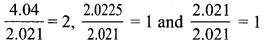Hence, the ratio of number of moles of 2 : 1 : 1 for H : C : Cl.
In case the ratios are not whole numbers, then they may be converted into whole number by multiplying by the suitable coefficient.

Step V:
Write empirical formula by mentioning the numbers after writing the symbols of respective elements. CH2Cl is thus, the empirical formula of the above compound.

Step VI:
Writing molecular formula
a. Determine empirical formula mass: Add the atomic masses of various atoms present in the empirical formula.
For CH2Cl, empirical formula mass = 12.000 + 2 × 1.008 + 35.453 = 49.469 g mol-1
b. Divide molar mass by empirical formula mass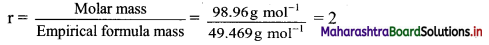c. Multiply empirical formula by r obtained above to get the molecular formula:
Molecular formula = r × empirical formula
∴ Molecular formula is 2 × CH2Cl i.e. C2H4Cl2.
Ans: The empirical formula of the compound is CH2Cl and the molecular formula of the compound is C2H4Cl2.
[Note: The question is modified to include the determination of molecular formula of the compound.]

Question 42.
A compound with molar mass 159 was found to contain 39.62% copper and 20.13% sulphur. Suggest molecular formula for the compound (Atomic masses: Cu = 63, S = 32 and O = 16).
Solution:
Given: Atomic mass of Cu = 63, S = 32, and O = 16
Percentage of copper and sulphur = 39.62% and 20.13% respectively.
To find: The molecular formula of the compound
Calculation: % copper + % sulphur = 39.62 + 20.13 = 59.75
This is less than 100 %. Hence, compound contains adequate oxygen so that the total percentage of elements is 100%.
Hence, % of oxygen = 100 – 59.75 = 40.25%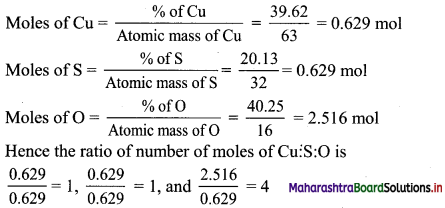Hence, empirical formula is CuSO4.
Empirical formula mass = 63 + 32 +16 × 4 = 159 g mol-1
Hence,
Molar mass = Empirical formula mass
∴ Molecular formula = Empirical formula = CuSO4
Ans: Molecular formula of the compound = CuSO4

Question 43.
An inorganic compound contained 24.75% potassium and 34.75% manganese and some other common elements. Give the empirical formula of the compound. (K = 39 u, Mn = 54.9 u, O = 16 u)
Solution:
Given: Atomic mass of K = 39 u, Mn = 59 u, and O = 16 u.
Percentage of potassium and manganese = 24.75 % and 34.75% respectively
To find: The empirical formula of the given inorganic compound
Calculation: Percentage of potassium = 24.75%
Percentage of manganese = 34.75%
Total percentage = 59.50%
∴ Remaining must be that of oxygen
∴ Percentage of oxygen = 100 – 59.50 = 40.50%
Moles of K = $$\frac{\% \text { of } \mathrm{K}}{\text { Atomic mass of } \mathrm{K}}=\frac{24.75}{39}$$ = 0.635 mol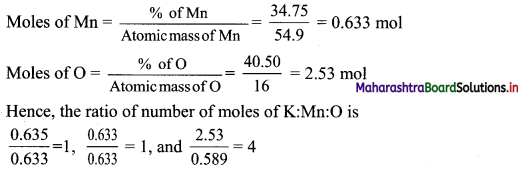Empirical formula = KMnO4
Ans: The empirical formula of given inorganic compound is KMnO4.Question 44.
An organic compound contains 40.92% carbon by mass, 4.58% hydrogen and 54.50% oxygen. Determine the empirical formula of the compound.
Solution:
Given: Percentage mass of carbon = 40.92%; Percentage mass of hydrogen = 4.58%
Percentage mass of oxygen = 54.50%
To find: The empirical formula of compound
Calculation: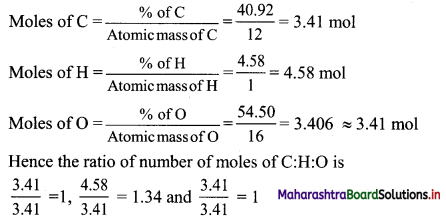∴ Ratio = 1 : 1.34 : 1
Multiply by 3 to get whole number
∴ Ratio = 3 : 4.02 : 3 ≈ 3 : 4 : 3
∴ The empirical formula of the compound is C3H4O3.
Ans: Empirical formula of the compound is C3H4O3.

Question 45.
Define stoichiometric calculations.
Calculations based on a balanced chemical equation are known as stoichiometric calculations.

Question 46.
Give reason: Balanced chemical equation is useful in solving problems based on chemical equations.
Balanced chemical equation is symbolic representation of a chemical reaction. It provides the following information, which is useful in solving problems based on chemical equations:

• It indicates the number of moles of the reactants involved in a chemical reaction and the number of moles of the products formed.
• It indicates the relative masses of the reactants and products linked with a chemical change, and
• It indicates the relationship between the volume/s of the gaseous reactants and products, at STP.

Hence, balanced chemical equation is useful in solving problems based on chemical equations.

Question 47.
What are different types of stoichiometric problems? Write steps involved in solving stoichiometric problems.
i. Generally, problems based on stoichiometry are of the following types:

• Problems based on mass-mass relationship
• Problems based on mass-volume relationship
• Problems based on volume-volume relationship.

ii. Steps involved in problems based on stoichiometric calculations:

• Write down the balanced chemical equation representing the chemical reaction.
• Write the number of moles and the relative masses or volumes of the reactants and products below the respective formulae.
• Relative masses or volumes should be calculated from the respective formula mass referring to the condition of STP.
• Apply the unitary method to calculate the unknown factors) as required by the problem.

Question 48.
Calculate the mass of carbon dioxide and water formed on complete combustion of 24 g of methane gas. (Atomic masses, C = 12 u, H = 1 u, O = 16 u)
Solution:
Mass of methane consumed in reaction = 24 g
Atomic mass: C = 12 u, H = 1 u, O = 16 u
To find: Mass of carbon dioxide and water formed
Calculation: The balanced chemical equation is,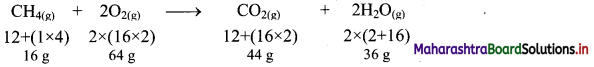Hence, 16 g of CH4 on complete combustion will produce 44 g of CO2.
∴ 24 g of CH4 = $$\frac {24}{16}$$ × 44 = 66 g of CO2
Similarly, 16 g of CH4 will produce 36 g of water.
∴ 24 g of CH4 = $$\frac {24}{16}$$ × 36 = 54 g of water
Ans: Mass of carbon dioxide and water formed respectively are 66 g and 54 g.Question 49.
How much CaO will be produced by decomposition of 5 g CaCO3?
Solution:
Given: Mass of CaCO3 consumed in reaction = 5 g
To find: Mass of CaO produced
Calculation: Calcium carbonate decomposes according to the balanced equation,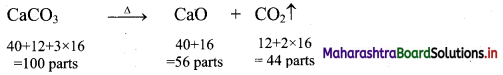So, 100 g of CaCO3 produce 56 g of CaO.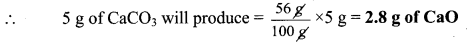Ans: Mass of CaO produced = 2.8 g

Question 50.
How many litres of oxygen at STP are required to burn completely 2.2 g of propane, C3H8 ?
Solution:
Given: Mass of propane used up in reaction = 2.2 g
To find: Volume of oxygen required at STP
Calculation:
The balanced chemical equation for the combustion of propane is,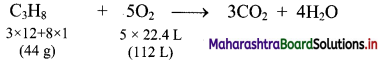(∵ 1 mol of ideal gas occupies 22.4 L of volume at STP)
Thus, 44 g of propane require 112 litres of oxygen at STP for complete combustion.
∴ 2.2 g of propane will require
$$\frac {112}{44}$$ × 2.2 = 5.6 litres of O2 at STP for complete combustion.
Ans: Volume of O2 (at STP) required to bum 2.2 g propane = 5.6 litres

Question 51.
A piece of zinc weighing 0.635 g when treated with excess of dilute H2SO4 liberated 200 cm3 hydrogen at STP. Calculate the percentage purity of the zinc sample.
Solution:
Given: Mass of zinc = 0.635 g, volume of H2 liberated = 200 cm3
To find: % purity of zinc sample
Calculation: The relevant balanced chemical equation is,
Zn + H2SO4 → ZnSO4 + H2
It indicates that 22.4 L of hydrogen at STP = 65 g of Zn.
(where, atomic mass of Zn = 65 u)
∴ 0.200 L of hydrogen at STP
= $$\frac{65 \mathrm{~g}}{22.4 \mathrm{~L}}$$ × 200 L
= 0.5803 g of Zn
∴ Percentage purity of Zn = $$\frac{0.5803}{0.635}$$ × 100
= 91.37 % (by using log tables)
Ans: Percentage purity of Zn sample = 91.37%

[Calculation using log table:
$$\frac{65 \times 0.200}{22.4}$$
= Antilog10 [log10 (65) + log10 (0.200) – log10 (22.4)]
= Antilog10 [1.8129 + $$\overline{1} .3010$$ – 1.3502]
= Antilog10 $\overline{1} .7637$ = 0.5803
$$\frac{0.5803}{0.635} \times 100=\frac{58.03}{0.635}$$
= Antilog10 [log10 (58.03) – log10 (0.635)]
= Antilog10 [1.7636 – $$\overline{1} .8028$$]
= Antilog10 [1.9608] = 91.37]

Question 52.
Explain with the help of a chemical reaction how limiting reagent works.

• Consider the formation of nitrogen dioxide (NO2) from nitric oxide (NO) and oxygen.
2NO(g) + O2(g) → 2NO2(g)
• Suppose initially, we take 8 moles of NO and 7 moles of O2.
• To determine the limiting reagent, calculate the number of moles NO2 produced from the given initial quantities of NO and O2. The limiting reagent will yield the smaller amount of the product.
• Starting with 8 moles of NO, the number of NO2 produced is,
8 mol NO × $$\frac{2 \mathrm{~mol} \mathrm{NO}_{2}}{2 \mathrm{~mol} \mathrm{NO}}$$ = 8 mol NO2
• Starting with 7 moles of O2, the number of moles NO2 produced is,
7 mol O2 × $$\frac{2 \mathrm{~mol} \mathrm{NO}_{2}}{1 \mathrm{~mol} \mathrm{NO}}$$ = 14 mol NO2
• Since, 8 moles NO result in a smaller amount of NO2, NO is the limiting reagent, and O2 is the excess reagent, before reaction has started.

Question 53.
Urea [(NH2)2CO] is prepared by reacting ammonia with carbon dioxide.
2NH3(g) + CO2(g) → (NH2)2CO(aq) + H2O(l)
In one process, 637.2 g of NH3 are treated with 1142 g of CO2.
i. Which of the two reactants is the limiting reagent?
ii. Calculate the mass of (NH2)2CO formed.
iii. How much excess reagent (in grams) is left at the end of the reaction?
Solution:
i. If 637.2 g of NH3 react completely, calculate the number of moles of (NH2)2CO, that could be produced, by the following relation.
Mass of NH3 → Moles of NH3 → Moles of (NH2)2CO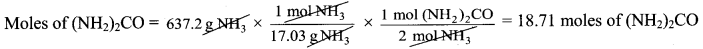If 1142 g of CO2 react completely, calculate the number of moles of (NH2)2CO, that could be produced, by the following relation.
Mass of CO2 → Moles of CO2 → Moles of (NH2)2CO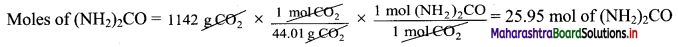Since NH3 produces smaller amount of (NH2)2CO, the limiting reagent is NH3.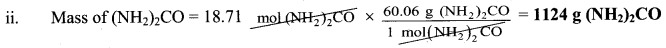iii. Starting with 18.71 moles of (NH2)2CO, we can determine the mass of CO2 that reacted using the mole ratio from the balanced equation and the molar mass of CO2 by the following relation.
Moles of (NH2)2CO → Moles of CO2 → Grams of CO2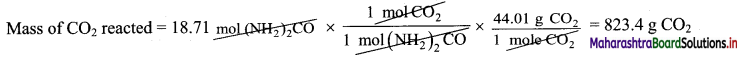The amount of CO2 remaining = 1142 g – 823.4 g = 318.6 g ≈ 319 g CO2 remaining
Ans: i. Limiting reagent = NH3
ii. Mass of (NH2)2CO produced = 1124 g
iii. Mass of CO2 remaining = 319 gQuestion 54.
6 g of H2 reacts with 32 g of O2 to yield water. Which is the limiting reactant? Find the mass of water produced and the amount of excess reagent left.
Solution:
i. The reaction is: 2H2(g) + O2(g) → 2H2O(l)
If 6 g of H2 react completely, calculate the number of moles of H2O, that could be produced, by the following relation.
Mass of H2 → Moles of H2 → Moles of H2O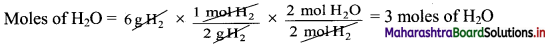If 32 g of O2 react completely, calculate the number of moles of H2O, that could be produced, by the following relation.
Mass of O2 → Moles of O2 → Moles of H2O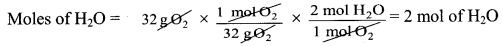Since O2 produces smaller amount of H2O, the limiting reagent is O2.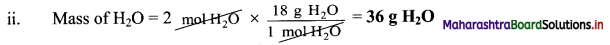iii. Starting with 2 moles of H2O, we can determine the mass of H2 that reacted using the mole ratio from the balanced equation and the molar mass of H2 by the following relation.
Moles of H2O → Moles of H2 → Grams of H2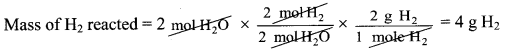The amount of H2 remaining = 6g – 4g = 2g H2 remaining
Ans: i. Limiting reagent = O2
ii. Mass of H2O produced = 36 g
iii. Mass of H2 remaining = 2 g

Question 55.
Name different ways to express the concentration of a solution (or the amount of substance in a given volume of solution).

• Mass percent or weight percent (w/w %)
• Mole fraction
• Molarity (M)
• Molality (m)

Question 56.
State the formula to obtain mass percent.
Mass percent (w/w %) = $$\frac{\text { Mass of Solute }}{\text { Massof solution }} \times 100$$

Question 57.
Why is molality NOT affected by temperature?

• Molality is the number of moles of solute present in 1 kg of solvent. Therefore, molality is mass dependent.
• Mass remains unaffected with temperature.

Hence, molality is not affected by temperature.

Question 58.
State TRUE or FALSE. Correct the statement if false.
i. A majority of reactions in the laboratory are carried out in in gaseous forms.
ii. Molarity is the most widely used to express the concentration of solution.
iii. Molality of a solution changes with temperature.
i. False
A majority of reactions in the laboratory are carried out in solution forms.
ii. Tme
iii. False
Molality of a solution does not change with temperature.

Question 59.
A solution is prepared by adding 2 g of a substance A to 18 g of water. Calculate the mass percent of the solute.
Solution:
Given: Mass of substance = 2 g, mass of water = 18 g
To find: Mass % of solute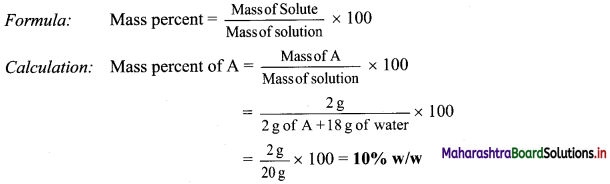Ans: Mass percent of A = 10 % w/wQuestion 60.
Calculate the molarity of NaOH in the solution prepared by dissolving its 4 g in enough water to form 250 mL of the solution.
Solution:
Given: Mass of solute (NaOH) = 4 g, volume of solution = 250 mL = 0.250 L
To find: Molarity of the solution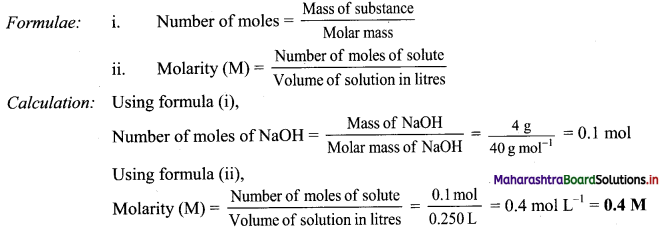Ans: Molarity of the NaOH solution = 0.4 M

Question 61.
The density of 3 M solution of NaCl is 1.25 g mL-1. Calculate molality of the solution.
Solution:
Given: Molarity of the solution = 3 M, density of the solution = 1.25 g mL-1
To find: Molality of the solution
Formula: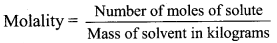Calculation: Molarity = 3 mol L-1
∴ Mass of NaCl in 1 L solution = 3 × 58.5 = 175.5 g
Mass of 1 L solution = 1000 × 1.25 = 1250 g (∵ Density = 1.25 g mL-1)
Mass of water in solution = 1250 – 175.5 = 1074.5 g = 1.0745 kg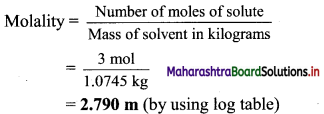Ans: Molality of the NaCl solution = 2.790 m

[Calculation using log table:
$$\frac{3}{1.0745}$$
= Antilog10 [log10 (3) – log10 (1.0745)]
= Antilog10 [0.4771 – 0.0315]
= Antilog10 [0.4456] = 2.790]

Question 62.
Calculate the molarity of 1.8 g HNO3 dissolved in 250 mL aqueous solution.
Solution:
Mass of HNO3 = 1.8 g,
volume of solution = 250 mL = 0.250 L
To find: Molarity
Formulae: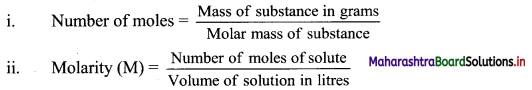Calculation: Molar mass of HNO3 = 63 g mol-1
Using formula (i),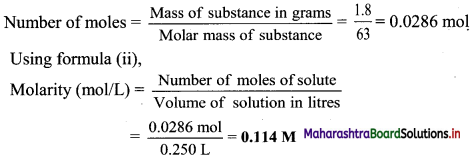Ans: Molarity of the HNO3 solution = 0.114 M

Question 63.
What volume in mL of a 0.1 M H2SO4 solution will contain 0.5 moles of H2SO4?
Solution:
Given: Molarity of H2SO4 solution = 0.1 M
Moles of H2SO4 = 0.5 mol
To find: Volume of H2SO4 solution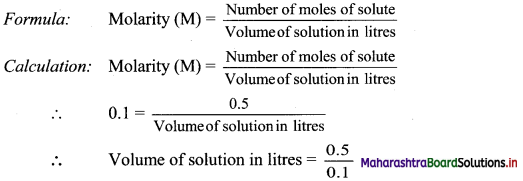= 5 L
= 5000 L
Ans: Volume of a 0.1 M H2SO4 solution = 5000 mLQuestion 64.
A mixture has 18 g water and 414 g ethanol. What are the mole fractions of water and ethanol?
Solution:
Given: Mass of water = 18 g, mass of ethanol = 414 g
To find: Mole fractions of water and ethanol
Formulae: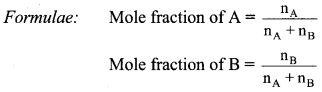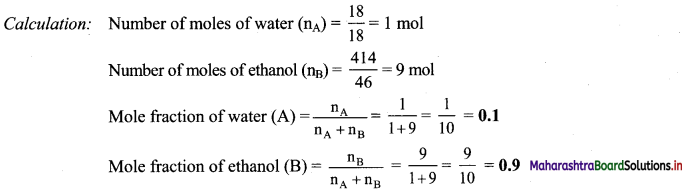Ans: Mole fraction of water = 0.1
Mole fraction of ethanol = 0.9

Question 65.
Explain in brief: Use of graphs in analytical chemistry.
i. Analytical chemistry often involves deducing some relation between two or more properties of matter under study.
ii. For example, the relation between temperature and volume of a given amount of gas.
iii. A set of experimentally measured values of volume and temperature of a definite mass of a gas upon plotting on a graph paper appears as in the figure below: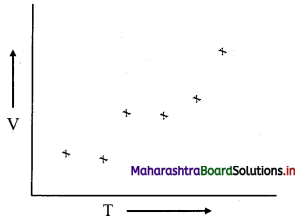iv. When the points are directly connected, a zig zag pattern results as shown below: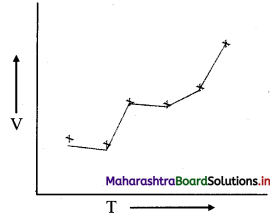From the above pattern, no meaningful result can be deduced.
v. A smooth curve (or average curve) passing through these points can be drawn as shown below. This straight line is consistent with the V ∝ T .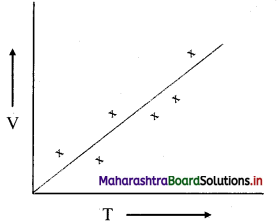vi. While fitting the points into a smooth curve, all the plotted points should be evenly distributed. This can be verified mathematically, by drawing a perpendicular from each point to the curve. The perpendicular represents deviation of each point from the curve. Take sum of all the perpendiculars on side of the line and sum of all the perpendiculars on another side of the line separately. If the two sums are equal (or nearly equal), the curve drawn shows the experimental points in the best possible representation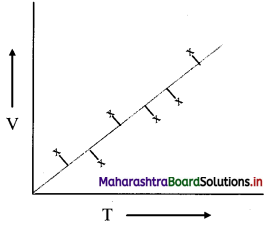Question 66.
1.5 g of an impure sample of sodium sulphate dissolved in water was treated with excess of barium chloride solution when 1.74 g of BaSO4 was obtained as dry precipitate. Calculate the percentage purity of the sample.
Solution: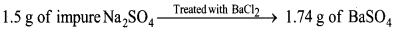The chemical equation representing the reaction is: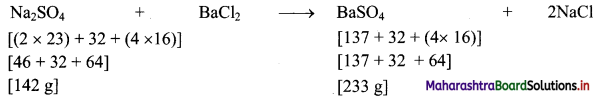To calculate the mass of Na2SO4 from which 1.74 g of BaSO4 is obtained:
233 g of BaSO4 is produced from 142 g of Na2SO4.
∴ Mass of Na2SO4 from which 1.74 g of BaSO4 would be obtained = $$\frac {142}{233}$$ × 1.74 = 1.06 g
∴ The mass of pure Na2SO4 present in 1.5 g of impure sample = 1.06 g
To calculate the percentage purity of the impure sample:
1.5 g of impure sample contains 1.06 g of pure Na2SO4
∴ 100 g of the impure sample will contain = $$\frac{1.06}{1.5}$$ × 100 = 70.67 g of pure Na2SO4
Ans: Percentage purity of the sample is 70.67 %.

Question 67.
Calculate the amount of lime Ca(OH)2, required to remove hardness of 50,000 L of well water which has been found to contain 1.62 g of calcium bicarbonate per 10 L .
Solution:
Calculation of total Ca(HCO3)2 present:
10 L of water contains 1.62 g of Ca(HCO3)
∴ 50,000 L of water will contain $$\frac{1.62}{10}$$ × 50,000 = 8100 g of Ca(HCO3)
Calculation of lime required:
The balanced equation for the reaction: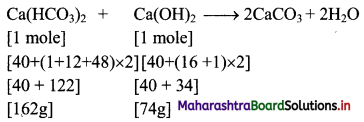∴ 162 g of Ca(HCO3) requires 74 g of lime.
Mass of lime required by 8100 g of Ca(HCO3) = $$\frac {74}{162}$$ × 8100 g = 3700 g = 3.7 kg
Ans: The amount of lime required to remove hardness of 50,000 L of well water, with 1.62 g of calcium bicarbonate per 10 L, is 3.7 kg.Multiple Choice Questions

1. The number of significant figures in 0.0110 is …………….
(A) 2
(B) 3
(C) 4
(D) 5
(B) 3

2. For the following measurements in which the true value is 4.0 g, find the CORRECT statement.

 Student Readings (g) A 4.01 3.99 B 4.05 3.95

(A) Results of both the students are neither accurate nor precise.
(B) Results of student A are both precise and accurate.
(C) Results of student A are neither precise nor accurate.
(D) Results of student B are both precise and accurate.
(B) Results of student A are both precise and accurate.

3. 18.238 is rounded off to four significant figures as ………….
(A) 18.20
(B) 18.23
(C) 18.2360
(D) 18.24
(D) 18.24

4. The % of H2O in Fe(CNS)3.3H2O is ……………..
(A) 34
(B) 11
(C) 19
(D) 46
(C) 19

5. The molecular mass of an organic compound is 78 g mol-1. Its empirical formula is CH. The molecular formula is ………….
(A) C2H4
(B) C2H2
(C) C6H6
(D) C4H4
(C) C6H6

6. The percentage of oxygen in NaOH is ……………
(A) 40%
(B) 60%
(C) 8%
(D) 10%
(A) 40%7. 1.2 g of Mg (At. mass 24) will produce MgO equal to …………….
(A) 0.05 mol
(B) 0.03 mol
(C) 0.01 mol
(D) 0.02 mol
(A) 0.05 mol

8. …………. reagent is the reactant that reacts completely but limits further progress of the reaction.
(A) Oxidizing
(B) Reducing
(C) Limiting
(D) Excess
(C) Limiting

9. If a solution is made up of 1 mol ethanol and 9 mol water, then mole fraction of water in the solution is ……………
(A) 0.1
(B) 0.5
(C) 0.9
(D) 1.0
(C) 0.9

10. 0.9 glucose (C6H12O6) is present in 1 L of solution. Find molarity.
(A) 5 M
(B) 50 M
(C) 0.005 M
(D) 0.5 M